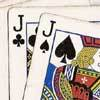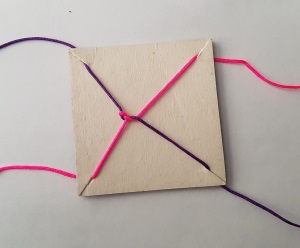#### You may also like### Do Unto Caesar

At the beginning of the night three poker players; Alan, Bernie and Craig had money in the ratios 7 : 6 : 5. At the end of the night the ratio was 6 : 5 : 4. One of them won $1 200. What were the assets of the players at the beginning of the evening?### Plutarch's Boxes According to Plutarch, the Greeks found all the rectangles with integer sides, whose areas are equal to their perimeters. Can you find them? What rectangular boxes, with integer sides, have their surface areas equal to their volumes?### 3388 Using some or all of the operations of addition, subtraction, multiplication and division and using the digits 3, 3, 8 and 8 each once and only once make an expression equal to 24. # Twisting and Turning ##### Age 11 to 14 Challenge Level: ### Why do this problem? This problem introduces an intriguing trick which provides a context for practising manipulation of fractions. Watching the video, or perhaps trying the trick out for themselves, can engage students' curiosity, and lead to some intriguing mathematics to explore and explain. ### Possible approach Show the video in the problem, and explain the two functions: Twisting has the effect of adding 1: $$x \mapsto x+1$$ Turning transforms any number into the negative of its reciprocal: $$x \mapsto -\frac1x$$ You may wish to pause the video at each stage and invite students to work out what the next fraction will be. Below is a useful table showing the operation and the fraction at each stage of the video. Operation Fraction Twist$1$Twist$2$Turn$-\frac12$Twist$\frac12$Twist$1\frac12$, or$\frac32$Twist$2\frac12$, or$\frac52$Turn$-\frac25$Twist$\frac35$Twist$1\frac35$, or$\frac85$Twist$2\frac35$, or$\frac{13}5$Turn$-\frac5{13}$If students have grasped the two operations, you could challenge them to work out a sequence of twists and turns to get back from$-\frac5{13}$to$0$. Alternatively, watch the rest of the video to see the surprising moment when the ropes disentangle. Here is the sequence: Operation Fraction Twist$\frac8{13}$Turn$-\frac{13}8$or$-1\frac58$Twist$-\frac58$Twist$\frac38$Turn$-\frac83$or$-2\frac23$Twist$-\frac53$or$-1\frac23$Twist$-\frac23$Twist$\frac13$Turn$-3$Twist$-2$Twist$-1$Twist$0\$

Now that students understand the two operations, set them the challenge in the problem - working out the fraction for each stage of the sequence, and working out the sequence to get back to zero:
Twist, twist, twist, turn, twist, twist, twist, turn, twist, twist, twist, turn

To finish off, students could perform their sequence to tangle and untangle their own set of skipping ropes, before moving on to More Twisting and Turning. Alternatively, they could perform the sequence individually using string and card, as shown below.### Key questions

What fraction do you reach next if you twist?
What fraction do you reach next if you turn?
How will you decide whether to twist or turn next?

### Possible extension

This is the first of three related problems. The second problem is More Twisting and Turning and the third is All Tangled Up.

### Possible support

For a more structured opportunity to practise operations with fractions, see Peaches Today, Peaches Tomorrow...